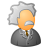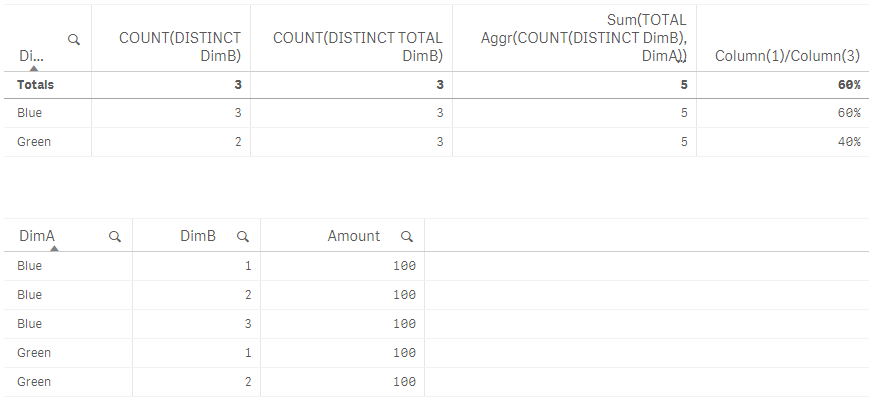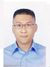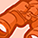Announcements
Applications are open for the 2024 Qlik Luminary Program. Apply by December 15 here.
cancel
Showing results for
Did you mean:Partner - Creator II

## Count total distinct

Hi everyone

Im working on some data that looks like this:

DimA, DimB, Amount

Blue, 1,  100

Blue, 2,  100

Blue, 3,  100

Green, 1, 100

Green, 2, 100

Count(Distinct DimB) results in barchart as

Blue, 3

Green, 2

And the formulae COUNT(DISTINCT TOTAL DimB) results in 3

If I try and get the right relative value for each dimension, so that

Blue = 3 / 5 = 60%

Green = 2 / 5 = 40%

But the formulae COUNT(DISTINCT DimB) / COUNT(DISTINCT TOTAL DimB)  results in =>

Blue 3/3 = 100%

Green 2/3 = 66%

I.e. the 3 / 5 should refer to the total sum of the rows.

In a bar chart how can I get the right relative value so that its based on the sum of the rows and not the count distinct?

Thank you

1 Solution

Accepted SolutionsEmployee

This should work.

Sum(TOTAL Aggr(COUNT(DISTINCT DimB), DimA))7 RepliesPartner - Creator II
Author

Tried this as well but came up with the wrong values

=SUM(TOTAL Aggr( Count(Distinct DimB), DimA ))Partner - Master III

COUNT(DISTINCT TOTAL DimB) will give you 3

COUNT( TOTAL DimB) will give you 5

Try

COUNT(DISTINCT DimB) / COUNT(TOTAL DimB)Employee

That wouldn't give the distinct count in the denominator. I would think the Aggr() might be the easiest way.Specialist

Hi Ruan,

As i can see  there are 3 distinct values of DimB on your data.

And this expression: COUNT(DISTINCT TOTAL DimB)  will return 3.

If you want the result is 5, try this: COUNT(TOTAL DimB)

Best.Employee

This should work.

Sum(TOTAL Aggr(COUNT(DISTINCT DimB), DimA))Partner - Creator II
Author

Thanks, I agree it makes sense,

but as I mentioned earlier it returns the wrong value.

Edit: I played around with the data I provided and this solution

did return the correct values, so I'll mark it as correct.

Not quite sure why it didn't apply to my data though.

Thanks for the help.

Will play around with the AGGR function more.Contributor II

Can you please provide the solution to this problem? I'm tryng to achieve the same result and have the same problem.

ThanksTags
Community Browser